## Motion Class 9 Notes:

Do you know, you can prepare for your exams easily and score better? Yes, of course. You simply have to gather revision material. To help you in doing so we have provided you with motion class 9 notes in this section. CBSE class 9 physics motion notes include distance, displacement, uniform and non-uniform motion, speed, velocity, acceleration, equations and graphical representation of motion and also uniform circular motion.

## Rest:

• When the position of body doesn’t change with respect to time then body is said to be in
• Book on a table, mirror on the well etc. are the examples of rest.
• Position is the location of any object or thing.

## Motion:

• When the position of body continuously changes with respect to time and a location.
• Distance and time are important factors to consider motion.

## Types of motion:

On the basis of path followed by any object, in motion class 9 notes, the motion are of three types:

1. Straight line motion:

• When there is movement of particle in space from one point to other along straight path, then it is called straight line motion (translatory motion)
• Motion of car on road is an example of straight line motion.
• We

2. Circular motion:

• Motion of an object along a circular path is said to be circular motion.
• Motion of satellite around earth is the best example of circular motion.
• Here, motion class 9 notes will give you a better way to revise NCERT books.

3. Vibratory motion/ Oscillatory motion:

• When objects move to and fro with respect to a particular position is said to be vibratory motion.
• Motion of pendulum is an example of oscillatory or vibratory motion.

## Scalar and Vector Quantities:

• Scalar quantity: When any physical quantity has only magnitude, not direction, then it is called scalar quantities. Mass, length, time etc. are scalar quantities.
• Vector Quantity: Those physical quantities which has both magnitude, as well as direction, are said to be vector quantities. Force, velocity, position are vector quantities.

## Distance

• The length of the actual path covered by any object is said to be
• It is scalar quantity.
• Example: Ria travelled 8 km from her bike.

## Displacement

• The shortest distance between the starting point and the end point is said to be displacement.
• It is vector quantity.
• Example: Ria travelled 8km due east.
• In some cases, displacement can be zero while you have travelled some distance. The case results when initial and final point of travel is same.

### Difference between distance and displacement:

 Distance Displacement Length of actual path covered byany body. Shortest length between initialand final position. Scalar quantity Vector quantity Distance is always positive. Displacement can be positivenegative or zero. Distance is always greater than or equal to displacement. Displacement is always less than or equal to distance.

### Types of motion on the basis of time frame:

There are two types of motion on the basis of time frame. In motion class 9 notes, they are:

• Uniform Motion
• Non-uniform Motion

## Uniform Motion:

• When an object travels equal distances in equal interval of time is said to be uniform motion.
• Example: A vehicle moving with constant velocity over a straight path.

## Non-Uniform Motion:

• When a body covers unequal distances in equal interval of time are said to be non-uniform motion.
• Example: When a bike is moving in a high and low traffic road one after the other then their velocity doesn’t remain same.

## Speed:

• The distance covered by a body per unit time is said to be
• Speed = $$\frac{Distance\: Travelled}{Time\:taken}$$
• The SI unit of speed is m/s.
• It is scalar quanity.

## Average Speed:

• The ratio of total distance travelled by a body to the time taken is its average speed.
• Average speed = $$\frac{Total\: distance\:travelled}{Total\: time\:taken}$$
• Its unit is also m/s.
Read motion class 9 notes wisely to score better in examination.

## Velocity:

• Displacement of a body per unit time is said to be
• We can also say that speed with a specific direction.
• Velocity = $$\frac{displacement}{time}$$
• Its Unit is also m/s.
• It is a vector quantity.

### Difference between Speed and velocity:

 Speed Velocity Distance per unit time. Displacement per unit time. It is scalar quantity. It is vector quantity. Speed has no direction. Velocity has direction asof displacement.

## Average velocity:

• The ratio of total displacement to that total time taken to cover the same is said to be average velocity.
• The average velocity of a body is always greater than average velocity in a straight path.
• If body returns to the same point where it has started then average velocity will be zero but average speed will not be equal to zero.
• For non-uniform motion, Average velocity(Vavg)= $$\frac{Total\:displacement}{Total\:time}$$
• For uniform motion, If initial velocity = u and final velocity = v, Average velocity (Vavg) = $$\frac{v + u}{2}$$

### Instantaneous speed and Instantaneous velocity

• Instantaneous speed is the speed of a body at a particular instant.
• When an object is in motion then at a specific time the velocity of body is said to be instantaneous velocity.
• Motion class 9 notes will provide you with sufficient information about each topic.

## Acceleration:

• The change in velocity per unit time is said to be
• It is observed in non-uniform motion.
• Its SI unit is m/s2.
• It is a vector quantity.
• a = $$\frac{Final\:velocity\:-\:Initial\:velocity}{Time}$$
• If initial velocity is represented by ‘u’, final velocity by ‘v’ and time by ‘t’ then acceleration ‘a’ is given by:
a = $$\frac{v-u}{t}$$
• As velocity increases with time, the acceleration also follows the direction of velocity.
• But if velocity decreases with time then acceleration follows the opposite direction of velocity.
• Negative acceleration is called retardation. It happens when velocity of a body decreases.
• Read acceleration and velocity variation further in motion class 9 notes.

### Uniform acceleration and Non-uniform acceleration:

• Uniform acceleration is when the body changes its velocity equally in equal intervals of time.
Example: Free falling of body from upwards.
• Non-uniform acceleration is when the body changes its velocity in unequal amounts in equal intervals of time.
Example: Motion of a vehicle.

## Equation of uniformly accelerated motion:

In uniformly accelerated motion displacement, velocity, time have special relations. Take a look at it as included in motion class 9 notes.

 Terms Symbols Displacement S Time t Initial Velocity u Final Velocity v Acceleration a

Equations are:

• v = u + at
• s = ut + $$\frac{1}{2}$$at2
• v2 = u2 + 2as

## Graphical representation of motion:

In motion, class 9 notes graphical representation is the most important part. Look at each graph and apply the equations to verify that they exist for that situation or not.

### Distance-time graph:

 Criteria of Graph properties Graph Body at rest Slope is zero,so speed = 0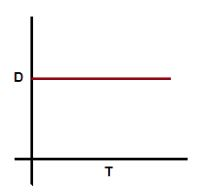Body moving withuniform speed Slope of graph = 1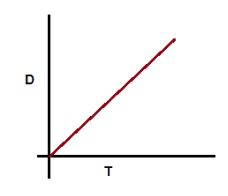For acceleratedmotion Slope increases with time.For Retardingbody Slope decreaseswith time.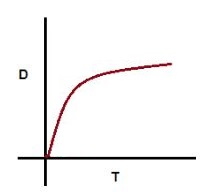### Velocity-time graph:

These graphs will help you to understand the topics of motion class 9 notes clearly. Take a look at it.

 Criteria of Graph properties Graph Body moving withuniform velocity Slope shows zero acceleration.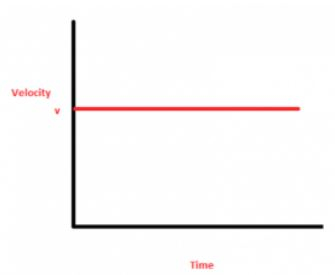Body starting from rest and moves withuniform acceleration. Greater slope carries greateracceleration.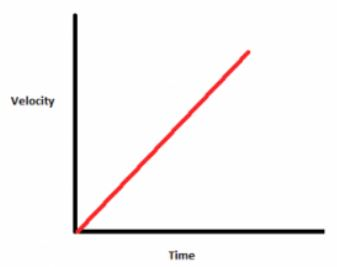Body moving with uniform accelerationbut initial velocity is non-zero. Graph shiftson y-axis.Body moving withincreasing acceleration. Slope increaseswith time.Body moving with decreasing acceleration. Slope decreaseswith time.With non-zero initialvelocity,body moving with a uniform retardation Here, θ > 90°, graphhas a negative slope.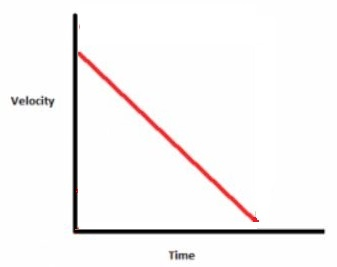## Uniform Circular Motion:

• When a body is moving on a circular path and with uniform speed is said to be uniform circular motion.
• Velocity of the body changes its direction at each and every point of
• Speed of the body may remain same at every point.

You can save a lot of time during revision. Motion class 9 notes are the complete revision material for the same. Whatever the material is required for your revision, it is thoroughly available in Motion 9th class note.